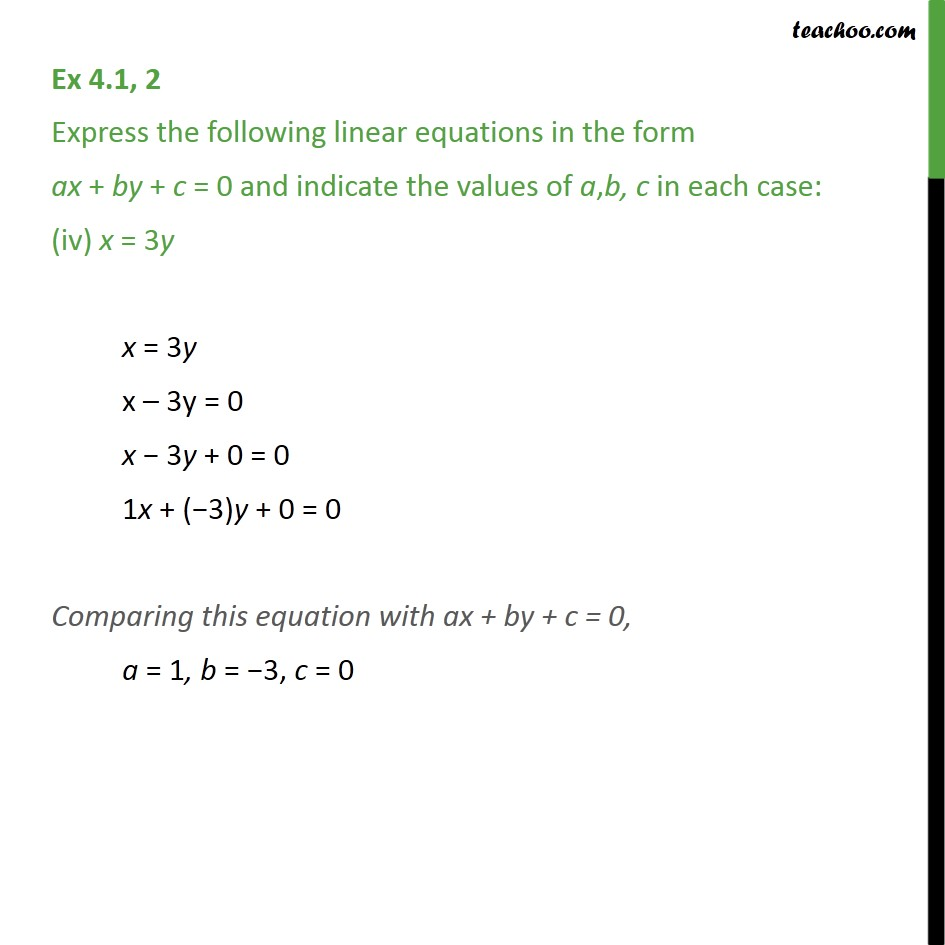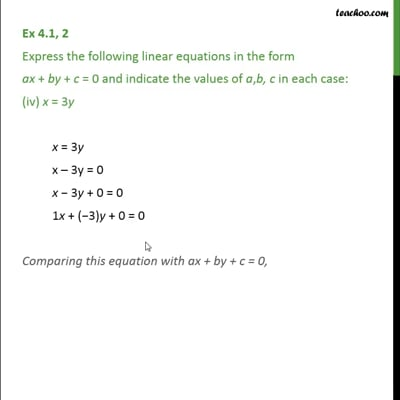Ex 4.1

Chapter 4 Class 9 Linear Equations in Two Variables
Serial order wiseThis video is only available for Teachoo black users

Get live Maths 1-on-1 Classs - Class 6 to 12

### Transcript

Ex 4.1, 2 Express the following linear equations in the form ax + by + c = 0 and indicate the values of a,b, c in each case: (iv) x = 3y x = 3y x – 3y = 0 x − 3y + 0 = 0 1x + (−3)y + 0 = 0 Comparing this equation with ax + by + c = 0, a = 1, b = −3, c = 0